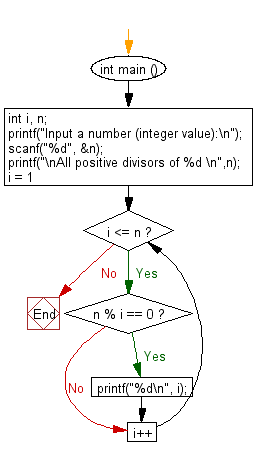﻿ C Program: Read an integer and compute all its divisors - w3resource

# C Exercises: Read an integer and compute all its divisors

## C Basic Declarations and Expressions: Exercise-121 with Solution

Write a C program that reads an integer and finds all the divisors of the said integer.

Sample Solution:

C Code:

``````#include <stdio.h>

int main () {
int i, n;

// Prompt user for input
printf("Input a number (integer value):\n");

// Read an integer value 'n' from user
scanf("%d", &n);

// Print a message indicating what the program will do
printf("\nAll positive divisors of %d \n",n);

// Loop through numbers from 1 to 'n'
for (i = 1; i <= n; i++) {

// Check if 'i' is a divisor of 'n'
if (n % i == 0) {
printf("%d\n", i); // Print 'i' if it is a divisor of 'n'
}
}

return 0; // End of program
}
``````

Sample Output:

```Input a number (integer value):
35

All positive divisors of 35
1
5
7
35
```

Flowchart:C programming Code Editor:

What is the difficulty level of this exercise?

Test your Programming skills with w3resource's quiz.

﻿